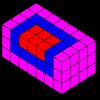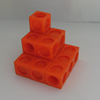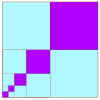Search by Topic

Resources tagged with Summation of series similar to Triangular Triples:

Filter by: Content type:
Age range:
Challenge level:

There are 10 results

Broad Topics > Patterns, Sequences and Structure > Summation of seriesSumming Squares

Age 14 to 16 Challenge Level:

Discover a way to sum square numbers by building cuboids from small cubes. Can you picture how the sequence will grow?Slick Summing

Age 14 to 16 Challenge Level:

Watch the video to see how Charlie works out the sum. Can you adapt his method?Picturing Square Numbers

Age 11 to 14 Challenge Level:

Square numbers can be represented as the sum of consecutive odd numbers. What is the sum of 1 + 3 + ..... + 149 + 151 + 153?Vanishing Point

Age 14 to 18 Challenge Level:

How can visual patterns be used to prove sums of series?The Root of the Problem

Age 14 to 18 Challenge Level:

Find the sum of this series of surds.Picture Story

Age 14 to 16 Challenge Level:

Can you see how this picture illustrates the formula for the sum of the first six cube numbers?Double Trouble

Age 14 to 16 Challenge Level:Sums of Powers - A Festive Story

Age 14 to 18

A story for students about adding powers of integers - with a festive twist.Pocket Money

Age 11 to 14 Challenge Level:

Which of these pocket money systems would you rather have?Summing Geometric Progressions

Age 14 to 18 Challenge Level:

Watch the video to see how to sum the sequence. Can you adapt the method to sum other sequences?